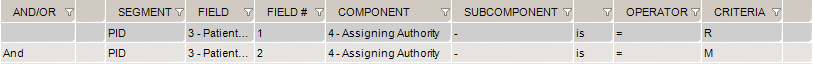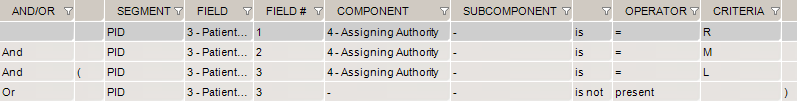# Validate Field repetition = value

## How to build a Segment/Field rule validating a specific field repetition has an expected value

In this example, let’s validate the following:

• the first repetition of PID.3.4 = R
• the second repetition of PID.3.4 = M
• the third repetition = L
• also, let’s assume the third repetition is optional and might not be provided
1. First set up your suite, scenario, and action.
2. In the inbound HL7 task, select the Validation tab
3. Select the Segment/Field Validation tab
4. Click Advanced Mode.  It reveals a few new columns.  The column FIELD # is where you set which repetition the rule applies to.
5. Add the first rule:  PID.3.4 (Field #1) is = R
6. Add a second rule: PID.3.4 (Field #2) is = M7. Now, let’s add 2 rules for the 3rd repetition. Remember that we need to account for cases where the 3rd repetition is not provided.
• Add the rule PID.3.4 (Field #3) is = L
• Add a last rule PID.3 (Field #3) is not present
• Either rule must be true so we add parentheses around the rules and use the OR logical operator